American Journal of Science, Engineering and Technology
Volume 1, Issue 2, December 2016, Pages: 63-67

Comparison of Methods for Calculation of Combustion Heat of Biopolymers

Michael Ioelovich

Designer Energy Ltd, Rehovot, Israel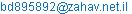Michael Ioelovich. Comparison of Methods for Calculation of Combustion Heat of Biopolymers. American Journal of Science, Engineering and Technology. Vol. 1, No. 2, 2016, pp. 63-67. doi: 10.11648/j.ajset.20160102.18

Received: November 18, 2016; Accepted: December 28, 2016; Published: January 16, 2017

Abstract: In this paper various methods for calculation of gross (Q) and net (q) heats of combustion for different biopolymers (lignin, cellulose, hemicelluloses, starch, pectin, proteins, lipids etc.) have been analyzed. The results showed that the calculation with use the energy released by combustion per gram of diatomic oxygen (Eq-parameter) is less accurate, because it gives a deviation from experimental values of about 4% for Q and more than 7% for q. In the case of calculations based on contribution of structural groups of polymers, the deviation may reach 3%. The lowest deviation of about 0.5% for Q and less than 1% for q was obtained using an improved method of calculation, which is based on elemental composition of the polymers. Calculation of gross and net heat of combustion for biomass samples by the improved method was very close to experimental calorific values. It was found that combustion of biomass waste supplemented with waste plastic is preferable, since such combustion technology provides more thermal energy than single firing of biomass and is accompanied by less emission of carbon dioxide in comparison with separate burning of plastic waste only.

Keywords: Biopolymers, Biomass, Plastics, Chemical Structure, Composition, Heat of Combustion, Calorimetry,
Calculation Methods

Contents

1. Introduction

Mankind produces a huge amount of solid waste including several billion tons of plant-based waste (paper, cardboard, textile, sawdust, shavings, branches, straw, husk, etc.) and hundreds million tons of waste plastics annually [1, 2]. Currently, main population of the world living in underdeveloped and developing countries, especially in rural areas, uses solid residues and wastes of the plant biomass (straw, bushes, twigs, firewood, sawdust, etc.) to meet their energy needs . In many countries, these resources account for over 90% of household energy consumption. In developed countries about 20% of solid organic waste is burned to generate the thermal energy . The not-edible plant materials are related to abundant, renewable and inexpensive source of the solid biofuel. To improve fuel properties, it is preferable to use the pelletized form of plant material containing high-energy binders, such as plastics, rubbers, tars, waxes, lipids, etc. [1, 5].

In order to determine the true value of gross energy or enthalpy of combustion of organic materials, a special apparatus is required such as precise, but complex and expensive calorimeter. Moreover, the calorimetric experiments are long and require the introduction of various corrections and laborious calculations. Therefore to characterize fuel properties of organic substances, e.g. combustible waste, the approximate calculations of calorific value are performed using equations of Mendeleev or other researchers [6, 7]. Estimating calculation method of combustion heat based on contribution of atoms and structural groups of polymers to thermal energy is also proposed . Study of 49 synthetic polymers showed that the net heat (Eq, kJ) released by combustion per gram of diatomic oxygen has approximately constant value: Eq= 13.10 ± 0.78 kJ per 1gr of O2 [9, 10]. Then, net heat of combustion (q, kJ/g) of the polymer sample can be calculated by the equation:

q = Eq nO2MO2/Mp (1)

where MO2 = 32 is molecular weight of O2 and nO2 is number of O2 molecules consumed for complete combustion of one mole of the repeat polymer unit having molecular weight Mp.

As known, vegetable biomass is a complex of natural biopolymers such as cellulose, hemicelluloses, lignin, starch, pectin, proteins, lipids and some others. Therefore, to estimate fuel properties of the biomass waste it is advisable to know beforehand the calorific value of the individual biopolymers. For this purpose it is necessary to perform a comparative analysis of various methods for calculating the combustion heat value of different biopolymers to choose the most accurate and reliable method.

2. Experimental

2.1. Materials

Various samples of biopolymers and plant biomass were studied (Tables 1 and 2).

Table 1. List of used biopolymers.

 Polymer Abbr Repeat unit Mp Glycine protein of wheat GLP C2H3O2N 73 Gluten of wheat GLT C5H6O2N 112 Xylan of birch XYL C5H8O4 132 Mannan of spruce MAN C6H10O5 162 Cellulose of cotton CEL C6H10O5 162 Starch of potatoes STR C6H10O5 162 Pectin of citrus PEC C6H8O6 176 Lignin of spruce LIG-S C10H11O3 179 Lignin of aspen LIG-A C11H12O4 208 Abietic acid ABA C20H30O2 302 Suberin of aspen bark SUB C72H140O6 1100

Note: Abbr is abbreviation; MW is molecular weight of repeat unit

Table 2. Chemical composition of biomass (wt. p.).

 Biomass Cellulose Hemi Lignin Extr Ash Softwood 0.48 0.20 0.28 0.02 0.02 Hardwood 0.45 0.25 0.23 0.03 0.04 Bagasse 0.40 0.31 0.20 0.03 0.06 Corn stover 0.38 0.33 0.2 0.02 0.07 Cardboard 0.60 0.12 0.18 0.03 0.07 Wr. paper 0.73 0.07 0.05 0.03 0.12

Note: Hemi is hemicelluloses; Extr is extractives; Wr is wrapping

2.2. Methods

Gross combustion heat (Q) of the samples was determined using a stainless steel calorimetric bomb having volume 0.320 dm3 at oxygen pressure of 3.05 MPa with 1.00 cm3 of deionized water added to the bomb . The combustion measurements were carried out by an isothermal water calorimeter at 298.15 K with accuracy ±0.001 K. The value of the energy equivalent of the calorimeter determined by standard benzoic acid was 15802.3 ± 0.9 J/K. The true mass of the sample used in each experiment was determined from the mass of the produced CO2. The system was thermostated for 1 h. After ignition, the temperature rise was measured within 1 h. All needed corrections were introduced. Conventional procedure was used to adjust the experimental combustion energy to standard conditions: T=298.15 K and p= 0.1 MPa. For each sample five experiments were performed to calculate an average value of combustion enthalpy and standard deviation.

The net combustion heat (q) of the samples was calculated by the equation :

q (MJ/kg) = Q (MJ/kg) – 0.22 H          (2)

where H is percentage of hydrogen in the sample.

The experimental results were compared with Q and q values obtained by various calculation methods based on equations of Mendeleev (eq. 3 and 4) , Parikh et al. (eq. 5 and 6) , improved eq. 7 and 8, on Eq-parameter (eq. 1 and 9) [9,10], as well as on structural groups of polymers .

Q (MJ/kg) = a1 C + b1 H – c1 O – d1 N – e1 A (3)

q (MJ/kg) = Q – f1 H (4)

where a1=0.339; b1=1.255; c1=0.109; d1=0.015; e1=0.02; f1 =0.226

Q (MJ/kg) = a2 C + b2 H – c2 O – d2 N – e2 A (5)

q (MJ/kg) = Q – f2 H (6)

where a2=0.349; b2=1.178; c2=0.103; d2=0.015; e2=0.02; f2 =0.202

Q (MJ/kg) = a3 C + b3 H – c3 O – d3 N – e3 A (7)

q (MJ/kg) = Q – f3 H (8)

where a3=0.344; b3=1.217; c3=0.106; d3=0.015; e3=0.02; f3 =0.210

In eq. 3-8: C, H, O, N is percentage of atoms in dry organic material; and A is percentage of ash in the sample.

Gross heat value can be also calculated using the parameter Eq:

Q = k Eq nO2MO2/Mp (9)

where k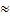1.075 is coefficient of transition from net to gross heat of combustion.

To implement the method of structural groups, the contributions of these groups to the heat of combustion should be used (Table 3).

Table 3. Structural groups and their contributions to gross heat of combustion .

 Group Qi, kJ/mol Group Qi, kJ/mol -CH3 775 -COO- 112 -CH2- 670 -C=O 259 -CH- 518 -NH- 77 -C- 431 -O- -132 -H 190 -OH -108

Gross heat of combustion was calculated by the equation:

Q = Σ ni Qi (10)

Then, the net combustion heat of the samples was calculated:

q = Q –f3 H (11)

The average relative deviation of calculated values from the experimental values was found by the equation:

RD (%) = 100 Σ(MSD/Xe)/n (12)

where the mean standard deviation is: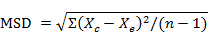Xc and Xe are the calculated and experimental values of combustion heat, respectively; n is the number of samples.

3. Results and Discussion

Results of experimental determination of gross and net heat of combustion for investigated biopolymers are shown in Table 4.

Table 4. Calorimetric data for gross (Q) and net (q) combustion heat of biopolymers.

 Polymer Formula Mp n(O2) Q, MJ/kg q, MJ/kg PEC C6H8O6 176 5 13.73 12.76 XYL C5H8O4 132 5 17.80 16.38 STR C6H10O5 162 6 17.53 16.23 MAN C6H10O5 162 6 17.50 16.20 CEL C6H10O5 162 6 17.45 16.12 GLP C2H3O2N 73 1.75 11.41 10.55 GLT C5H6O2N 112 5.5 21.87 20.64 LIG-S C10H11O3 179 11.25 27.70 26.45 LIG-A C11H12O4 208 12 25.72 24.83 ABA C20H30O2 302 26.5 38.25 36.17 SUB C72H140O6 1100 104 41.67 38.92

The experimental values of the combustion heat (EXP) were compared with the results of calculations using various methods (Tables 5 and 6). Analysis of the results showed that the calculations by eq. (1) and eq. (9) with use the Eq-parameter is less accurate and reliable because they give the relative deviation from experimental values of about 4% for Q and more than 7% for q. In the case of calculations by eq. (10) and eq. (11) based on contribution of structural groups of polymers, the deviation for some samples may reach 3%. The lowest deviation of about 0.5% for Q and less than 1% for q was obtained using an improved method of calculation by eq. (7) and (8), which is based on elemental composition of the polymers.

Table 5. Comparison of experimental and calculated values of gross combustion heat of biopolymers (Q, MJ/kg).

 Polymer EXP Eq. (3) Eq. (5) Eq. (7) Eq. (9) Eq. (10) PEC 13.73 13.56 13.93 13.75 12.80 13.70 XYL 17.80 17.67 18.00 17.81 17.07 17.14 STR 17.53 17.43 17.67 17.50 16.69 16.49 MAN 17.50 17.43 17.67 17.50 16.69 16.49 CEL 17.45 17.43 17.67 17.50 16.69 16.49 GLP 11.41 11.24 11.51 11.38 10.82 11.77 GLT 21.87 21.72 21.98 21.86 22.12 21.90 LIG-S 27.70 27.57 27.91 27.75 28.32 27.71 LIG-A 25.72 25.45 25.82 25.65 25.99 26.38 ABA 38.25 38.21 38.31 38.27 39.54 38.38 SUB 41.67 41.61 41.50 41.57 41.74 42.42 RD, % 0 1.4 0.8 0.5 4.0 2.9

Table 6. Comparison of experimental and calculated values of net combustion heat of biopolymers (q, MJ/kg).

 Polymer EXP Eq. (4) Eq. (6) Eq. (8) Eq. (1) Eq. (11) PEC 12.76 12.54 12.91 12.81 11.91 12.71 XYL 16.38 16.30 16.58 16.50 15.88 15.80 STR 16.23 16.00 16.23 16.21 15.52 15.18 MAN 16.20 16.00 16.23 16.21 15.52 15.18 CEL 16.12 16.00 16.23 16.21 15.52 15.18 GLP 10.55 10.31 10.69 10.52 10.04 10.86 GLT 20.64 20.51 20.80 20.74 24.33 20.71 LIG-S 26.45 26.17 26.52 26.45 26.35 26.34 LIG-A 24.83 24.15 24.51 24.43 25.18 25.10 ABA 36.17 35.98 36.10 36.10 36.78 36.30 SUB 38.92 38.76 38.62 38.90 38.83 39.63 RD, % 0 1.7 1.4 0.9 7.2 3.2

Calculation of gross and net heat of combustion for biomass samples was performed taking into account chemical composition of the samples (Table 2):

Q = Σ wi Qi                 (13)

q = Σ wi qi                 (14)

where wi is weight part of individual components of the biomass; Qi and qi are gross and net combustion heat calculated by the improved method (eq. 7 and 8).

As can be seen, the calculated heat values for various biomass samples were close to experimental values in the RD range of 1.0-1.2% (Table 7).

Table 7. Comparison of experimental (EXP) and calculated (CALC) values of combustion heat of biomass samples.

 Biomass Q, MJ/kg q, MJ/kg EXP CALC EXP CALC Softwood 20.45 20.53 19.11 19.18 Hardwood 19.70 19.44 18.35 18.14 Bagasse 19.00 18.84 17.82 17.60 Corn stover 18.37 18.46 17.44 17.58 Cardboard 18.48 18.75 17.21 17.50 Wr. paper 16.69 16.54 15.56 15.38

The main problem for using the biomass waste as solid fuel is relatively low heat output. One of the ways to solve this problem is to obtain a mixed solid fuel containing along with biomass waste also an additive of widespread waste plastics such as PE and/or PP. On the one hand it allows to release the environment from solid waste, and on the other hand the use of mixed fuel provides a greater amount of thermal energy (Fig. 1 and 2).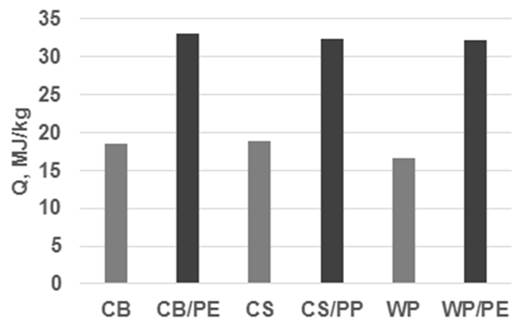Figure 1. Gross heat value of waste cardboard (CB), corn stover (CS), wrapping paper (WP) and mixture of these biomass waste with waste plastics (1:1).

Further more, since the biomass is neutral for emission of carbon dioxide into the environment, burning of the mixed fuel results in reduction of carbon dioxide emission by half in comparison with a separate burning of plastic waste only (Fig. 3).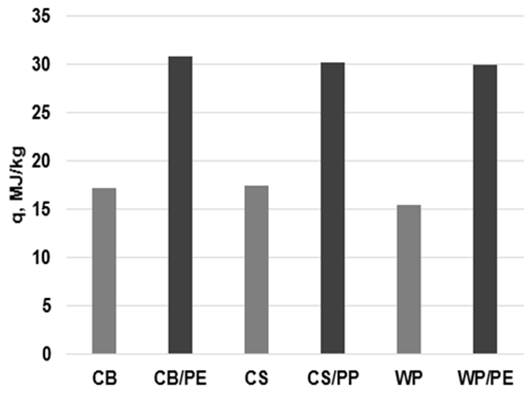Figure 2. Net heat value of waste cardboard (CB), corn stover (CS), wrapping paper (WP) and mixture of these biomass waste with waste plastics (1:1).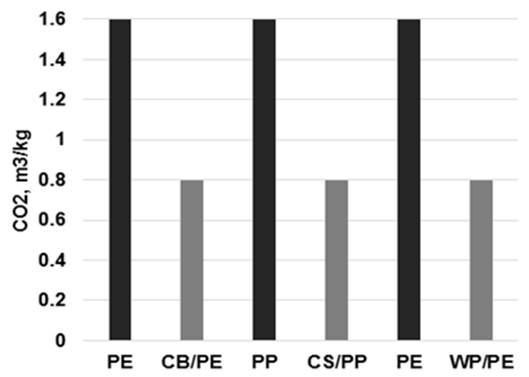Figure 3. Emission of carbon dioxide after burning of waste plastics and their mixture with biomass waste (1:1).

4. Conclusion

Vegetable biomass is a complex of natural biopolymers such as cellulose, hemicelluloses, lignin, starch, pectin, proteins, lipids and some others. Therefore, to estimate fuel properties of the biomass waste it is advisable to know beforehand the calorific value of the individual biopolymers. For this purpose a comparative analysis of various methods for calculating the combustion heat value of different biopolymers was carried out in order to choose the most accurate and reliable method.

Gross and net heats of combustion for different biopolymers such as lignin, cellulose, hemicelluloses, starch, pectin, proteins, lipids, as well as some biomass have been determined. The experimental values of the combustion heat were compared with the results of calculations using various methods. The results showed that the calculation with use the energy released by combustion per gram of diatomic oxygen (Eq-parameter) is less accurate and reliable because this method gives the relative deviation from experimental values of about 4% for Q and more than 7% for q. In the case of calculations based on contribution of structural groups of polymers, the deviation for some samples may reach 3%. The lowest deviation of about 0.5% for Q and less than 1% for q was obtained using an improved calculation method of gross (Q) and net (q) heat values, which is based on elemental composition of the polymers:

Q = 0.344 C + 1.217 H – 0.106 O – 0.015 N – 0.02A

q = Q – 0.210 H

where C, H, O, N is percentage of atoms in dry biopolymer; and A is percentage of ash in the sample.

Values of combustion heat for biomass samples calculated by the improved method were close to experimental calorific values. To generate the heat energy it is preferable to burn the biomass waste supplemented with the waste plastic, since such combustion technology provides more thermal energy than separate firing of biomass, as well as less emission of carbon dioxide in comparison with separate burning of plastic waste only.

References

1. Ioelovich M. "Problems of solid biofuels made of plant biomass," Advance in Energy, vol. 2 (1), pp. 15-20, 2014.
2. Ioelovich M. "Waste paper as promising feedstock for production of biofuel," J. Sci. Res. Report, vol. 3 (7), pp. 905-916, 2014.
3. Ioelovich M. Energetic Potential of Plant Biomass as a Renewable Source of Biofuels, in: Energy Science and Technology Series, Vol. 7: Bioenergy. Edited by R. Prasad, S. Sivakumar, U.C. Sharma. Studium Press LLC, Houston, 2015.
4. Energy recover from waste. Report of New Energy Co. http://www.newenergycorp.com.au/what-we-do/waste-hierarchy. New Energy, West Perth, 2016.
5. Ioelovich M. "Recent findings and the energetic potential of plant biomass as a renewable source of biofuels – a review," Bioresources, vol. 10 (1), pp. 1879-1914, 2015.
6. Suris A.L. "Heat of combustion of compounds," Chem. Pertol. Eng., vol. 43, pp. 20-21, 2007.
7. Parikh J., Channiwala S.A., Ghosal G.K. "A correlation for calculating HHV from proximate analysis of solid fuels," Fuel, vol. 84, pp. 487–494. 2005.
8. Walters R.N., Lyon R.E., Hackett S.M. "Heats of combustion of high-temperature polymers," Fire and Mater., vol. 24, pp. 1-13, 2000.
9. Huggett C. "Estimation of rate of heat release by means of oxygen consumption measurements," Fire and Mater., vol. 4, pp. 61-65, 1980.
10. Babrauskas V. Heat Release in Fires. Ch.8. Elsevier, London, 1992.
11. Ioelovich M. "Study of thermodynamic stability of various allomorphs of cellulose," J. Basic Appl. Res. Int., vol. 16, pp. 96-103, 2016.

 Contents 1. 2. 2.1. 2.2. 3. 4.
Article ToolsAbstractPDF(581K)